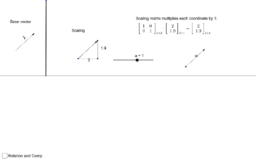•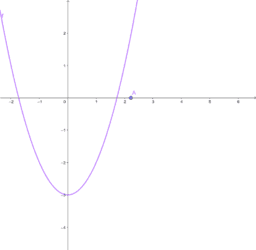Newton's Method

Activity

•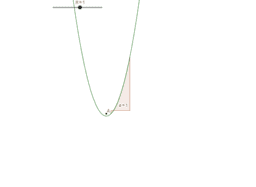Courtney

Activity

•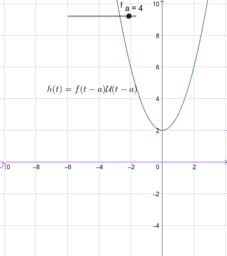Second translation theorem

Activity

•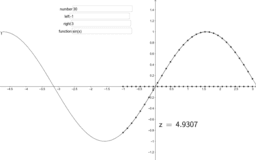Arc length

Activity

•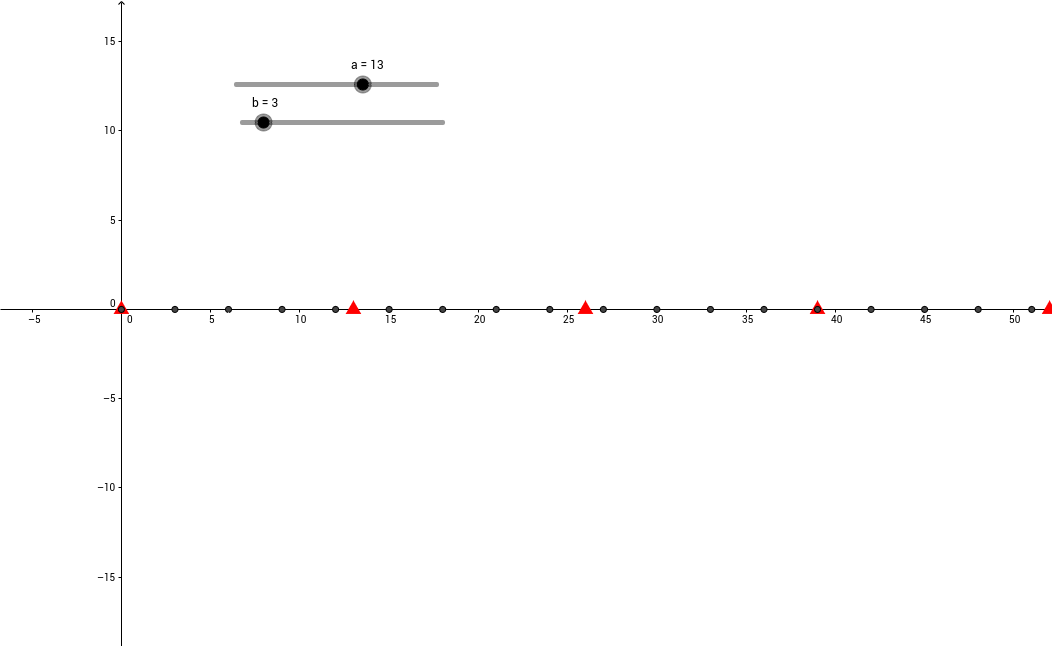LCM, Math 106

Activity

•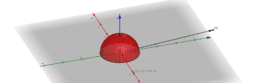Rayleigh quotient

Activity

•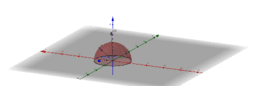Rayleigh quotient

Activity

•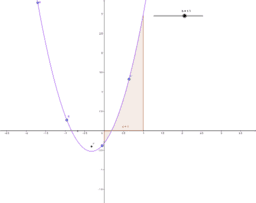Math 280 locus of focus

Activity

•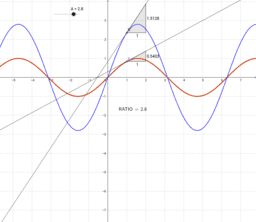Constant multiple rule

Activity

•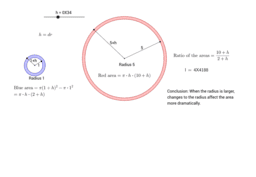Introduction to "Relative Change"

Activity

•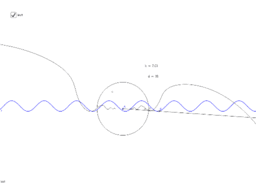Fun with College 101

Activity

•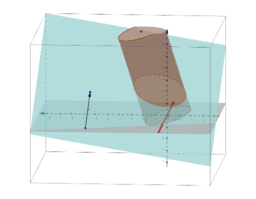cylinder insected by a place with given normal vector

Activity

•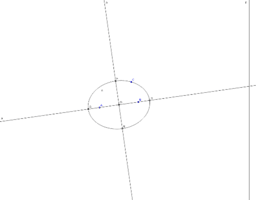Section of right circular cylinder

Activity

•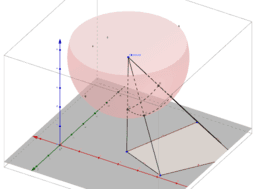project to sphere

Activity

•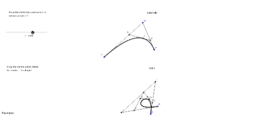Bezier construction

Activity

•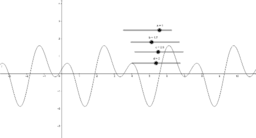Sines and sliders

Activity

•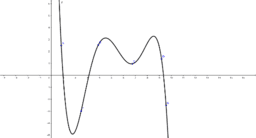Polynomial interpolation

Activity

•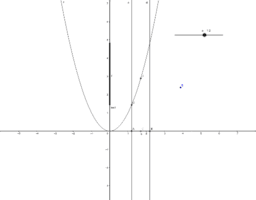Uniform Continuity

Activity

•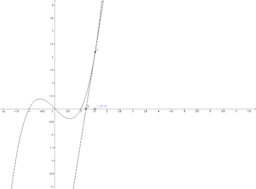Newton's Method

Activity

•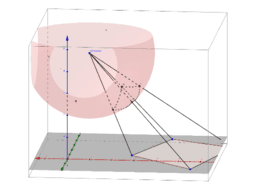Spherical perspective

Activity

•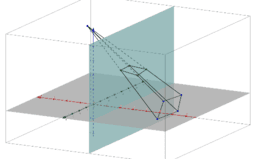Perspective

Activity

•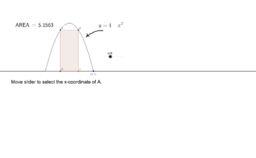Rectangle under a parabola

Activity

•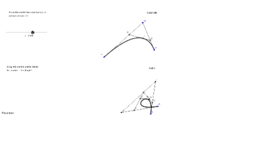Bezier construction

Activity

•